# MA.912.GR.6.2Export Print
Solve mathematical and real-world problems involving the measures of arcs and related angles.

### Clarifications

Clarification 1: Within the Geometry course, problems are limited to relationships between inscribed angles; central angles; and angles formed by the following intersections: a tangent and a secant through the center, two tangents, and a chord and its perpendicular bisector.
General Information
Subject Area: Mathematics (B.E.S.T.)
Strand: Geometric Reasoning
Status: State Board Approved

## Benchmark Instructional Guide

### Terms from the K-12 Glossary

• Central Angle
• Circle
• Inscribed Angle

### Vertical Alignment

Previous Benchmarks

Next Benchmarks

### Purpose and Instructional Strategies

In the middle grades, students encountered arc measure, arc length, and central angle by way of circle graphs and random experiments involving spinners, and they learned the formula for the circumference of a circle. In Algebra 1, students rearranged formulas to highlight a quantity of interest and solved linear equations in one variable. In Geometry, students learn a variety of results about angles and arcs in circles and how they relate to one another. In later courses, students will determine the value of trigonometric functions for real numbers by identifying angle measures in the unit circle.
• Instruction includes using precise definitions and language when working with angle measures and arcs involving circles. Students should be able to determine the similarities and differences between each of the various angle measures and arcs and how their relationships interact with one another. (MTR.4.1
• For example, students should be able to answer questions like “What is the difference between central angle and inscribed angle?”, “Does the diameter have a central angle?” and “What is the difference between the measure of an inscribed angle and the measure of an intercepted arc?”
• Instruction includes student understanding of the Inscribed Angle Theorem and how it relates to angle measures within a circle. Proving this Theorem requires three cases: (1) the center of the circle is on one of the chords, so one of the chords is a diameter; (2) the center of the circle is between the two chords; and (3) the center of the circle is not between the two chords. Below describes the proof of the first case of the Inscribed Angle Theorem.
• For example, given Circle C with the chord AB and the diameter AD, the central angle is BCD and the inscribed angle is DAB, which is the same as CAB. Students should realize that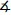BCD +BCA = 180. Students should also realize thatCAB +ABC +BCA = 180. Therefore, after using the Substitution Property of Equality,BCD +BCA =CAB +ABC +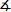BCA which is equivalent toBCD =CAB +ABC. Since two of the sides of triangle ABC are radii, students can classify it as an isosceles triangle, thereforeCAB =ABC. Students can use this fact to make the equivalent equationBCD =CAB +CAB which is equivalent toBCD = 2CAB. This equation proves that the central angle, angle BCD, has twice the measure of the inscribed angle, angle CAB, which is the first case of the Inscribed Angle Theorem.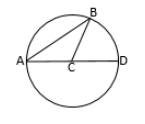• Instruction includes the connection to coordinate geometry to prove, or justify, that every angle inscribed in a semicircle is a right angle.
• For example, given Circle C below with chords AB and BD, students can use slope criteria to prove that angle ABD is a right angle.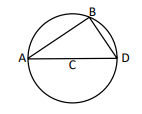• Instruction includes student understanding of the following relationships between angle measures and arcs in a circle (even though some extend beyond Clarification 1). Students should understand that most relationships between angles in a circle, and relationships between segments in a circle, can be derived from the Inscribed Angle Theorem. (MTR.5.1)
• The measure of a central angle is equal to the measure of its intercepted arc.
• The measure of an inscribed angle is half the measure of its intercepted arc.
• The measure of an inscribed angle is half the measure of the central angle that intercepts the same arc on the circle.
• In a circle (or congruent circles), any two inscribed angles with the same intercepted arcs are congruent.
• The measure of an angle formed by two tangents, two secants or a secant and a tangent from a point outside the circle, is half the difference of the measures of the intercepted arcs.
• The angle made by two intersecting tangents to a circle is called a circumscribed angle and it is supplementary to the central angle intercepting the same arc.
• An angle formed by two intersecting chords and whose vertex is inside the circle equals one-half the sum of its intercepted arcs.
• An angle formed by a chord and a tangent, whose vertex is on the circle, is one- half its intercepted arc.
• If one chord of a circle is a perpendicular bisector of another chord, then the first chord is a diameter and bisects the arc intercepted by the chord.
• Instruction includes the understanding and naming of major, minor and semicircle arcs.
• Problem types include determining missing angle measures in both mathematical and real-world contexts.
• Instruction includes the connection to arc lengths in circles. (MA.912.GR.6.4)

### Common Misconceptions or Errors

• Students may try to apply properties of central angles and their intercepted arcs to inscribed angles and intercepted arcs.
• Students may confuse arc measure and arc length, and may try to measure arcs with linear units rather than degrees.

• Find the measure of angle E in circle A.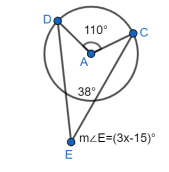Instructional Task 2 (MTR.2.1, MTR.4.1, MTR.5.1

• A circle is given below with two intersecting secants, PA and PC.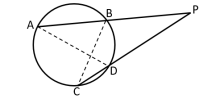• Part A. What is the sum of the measures of angle BCP, angle CPB and angle PBC
• Part B. What is the sum of the measures of angle PBC and angle ABC
• Part C. What can you conclude about the relationship between the sum of the measures of the three angles from Part A and the sum of the measures of the two angles from Part B?
• Part D. Using the information from Part C, what can you conclude about the measure of angle CPB? State your conclusion algebraically as an equation whereCPB =?.
• Part E. How can you use the information from Part D, to justify the Secant-Secant Angle Theorem which states that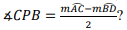### Instructional Items

Instructional Item 1
• The International Space Station (ISS) passes over the earth 248 miles above the earth’s surface. The angle formed between the two tangents formed from the ISS and the earth measures 140.4 °. What is the measure of the arc of the earth that could have a view of the ISS passing overhead?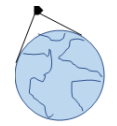*The strategies, tasks and items included in the B1G-M are examples and should not be considered comprehensive.

## Related Courses

This benchmark is part of these courses.
1200400: Foundational Skills in Mathematics 9-12 (Specifically in versions: 2014 - 2015, 2015 - 2022, 2022 and beyond (current))
1206310: Geometry (Specifically in versions: 2014 - 2015, 2015 - 2022, 2022 and beyond (current))
1206320: Geometry Honors (Specifically in versions: 2014 - 2015, 2015 - 2022, 2022 and beyond (current))
1206315: Geometry for Credit Recovery (Specifically in versions: 2014 - 2015, 2015 - 2022, 2022 and beyond (current))
7912065: Access Geometry (Specifically in versions: 2015 - 2022, 2022 and beyond (current))

## Related Access Points

Alternate version of this benchmark for students with significant cognitive disabilities.
MA.912.GR.6.AP.2: Identify the relationship involving the measures of arcs and related angles, limited to central, inscribed and intersections

## Related Resources

Vetted resources educators can use to teach the concepts and skills in this benchmark.

## Formative Assessments

Central and Inscribed Angles:

Students are asked to describe the relationship between a central angle and an inscribed angle that intercept the same arc.

Type: Formative Assessment

Inscribed Angle on Diameter:

Students are asked to find the measures of two inscribed angles of a circle.

Type: Formative Assessment

Circles with Angles:

Students are given a diagram with inscribed, central, and circumscribed angles and are asked to identify each type of angle, determine angle measures, and describe relationships among them.

Type: Formative Assessment

Arc Length:

Students are asked to find the lengths of arcs in two different circles.

Type: Formative Assessment

## Lesson Plans

Seeking Circle Angles:

Students will start this lesson with a win-lose-draw game to review circle vocabulary words. They will then use examples on a discovery sheet to discover the relationships between arcs and the angles whose vertex is located on a circle, in the interior of the circle, and exterior to the circle. They will wrap up the lesson in a class discussion and questions answered on white boards.

Type: Lesson Plan

Seeking Circle Segments:

Students will start this lesson with a "Pictionary" game to review circle vocabulary terms. They will then use computers and GeoGebra to discover the relationships between portions of segments that intersect in the interior of the circle, and exterior to the circle. They will wrap up the lesson in a class discussion and consensus on rules (formulas).

Type: Lesson Plan

## MFAS Formative Assessments

Arc Length:

Students are asked to find the lengths of arcs in two different circles.

Central and Inscribed Angles:

Students are asked to describe the relationship between a central angle and an inscribed angle that intercept the same arc.

Circles with Angles:

Students are given a diagram with inscribed, central, and circumscribed angles and are asked to identify each type of angle, determine angle measures, and describe relationships among them.

Inscribed Angle on Diameter:

Students are asked to find the measures of two inscribed angles of a circle.

## Student Resources

Vetted resources students can use to learn the concepts and skills in this benchmark.

## Parent Resources

Vetted resources caregivers can use to help students learn the concepts and skills in this benchmark.